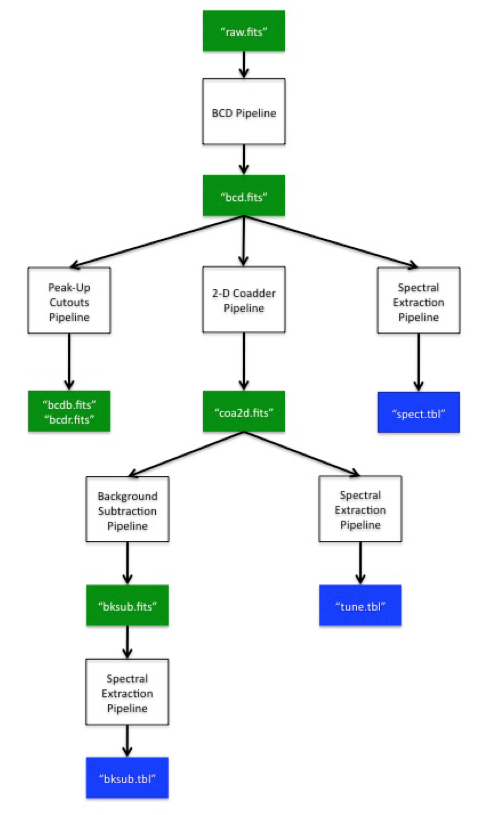Spitzer Documentation & Tools
IRS Instrument Handbook

# Chapter 5.    Pipeline Processing

This chapter describes the basic operation of the IRS pipelines, illustrated as a flow chart in Figure 5.1.  The following sections present descriptions of the modules comprising each pipeline: Sections 5.1 and 5.2 describe the BCD pipeline (Figure 5.2). Section 5.4 describes the Dark Calibration pipeline, which provides inputs to the BCD pipeline (Figure 5.9). Sections 5.5, 5.6, and 5.6 describe respectively the Peak-Up Cutouts, Two-Dimensional Coadder, and Background Subtraction pipelines. Section 5.7 describes the Spectral Extraction pipeline (Figure 5.10).

In what follows, an IRS data cube is a FITS file of dimensions 128×128×nplane, where nplane=4, 8, or 16 (depending on DCE integration time) for IRS Sample-Up-the-Ramp (SUR) data. The third axis in the cube can be interpreted as the time dimension. Each plane is a non-destructive read-out of the whole IRS array, and each pixel in a plane is referred to as a “sample.” A “ramp” is the vector of the 4, 8, or 16 samples of a particular pixel. That is, a “ramp” consists of the non-destructive read-outs of one pixel, ordered by increasing time. The term “read-out channel” in IRS refers to a particular set of columns of the array, consisting of every fourth column. The starting column in this set determines its IRS read-out channel designation. There are four IRS read-out channels: columns 1, 5, 9, etc. comprise IRS read-out channel 1; columns 2, 6, 10, etc. comprise IRS read-out channel 2, and so on.Figure 5.1  IRS Pipelines Flow chart. The BCD Pipeline is described in Sections 5.1 and 5.2, the Peak-Up Cutouts Pipeline is described in Section 5.4, the Two-Dimensional Coadder Pipeline is described in Section 5.5, the Background Subtraction Pipeline is described in Section 5.6, and the Spectral Extraction Pipeline is described in Section 5.7.